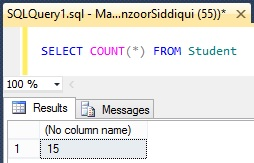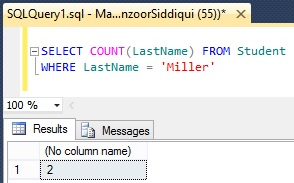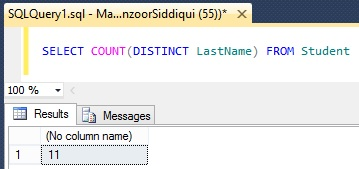# SQL COUNT() Function

SQL COUNT() function returns the number of records from a table in a SELECT statement.

## Syntax

SELECT COUNT (columnName) FROM TableName

OR

SELECT COUNT (*) FROM TableName

Note: Count(*) will return all the records from a table whereas COUNT(columnName) will return the number of values for specified column excluding NULL values.

Here we are going to use Student table for demonstration purpose.In the result pane you can see there are total 15 records in the table.

`SELECT COUNT(*) FROM Student `Now, we will take the count of LastName column as follows.

`SELECT COUNT(LastName) FROM Student`Count(LastName) has returned only 13 records which means that COUNT() function ignores NULL values in the specified column.

You can also use WHERE clause with COUNT() function to filter out the conditions as follows.

```SELECT COUNT(LastName) FROM Student
WHERE LastName = 'Miller'```We will take one more example for COUNT() function with DISTINCT clause.

`SELECT COUNT(DISTINCT LastName) FROM Student `As you can see in the output there are only 11 distinct records in LastName column.

Reference: Manzoor Siddiqui [www.SQLServerLog.com]